### Referencing Field Names across tables with Variables?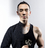I have two tables, HerbInventory and Client. HerbInventory just contains records of all the herbs I have, and I want to be able to prescribe herbs in the Client table. Currently what I have done is give the Client form 10 separate fields linked to HerbInventory table (named as Herb01, Herb02, Herb03 etc.) so they can input up to 10 herbs per client that is available from the HerbInventory table.

I want to create a button in the Client table form where it takes the value of the 10 fields (name of the herbs) and output it in one string variable. I can do this manually by writing Client.Herb01, Client.Herb02 and so on for each field. But if I had 100 fields, this code would be crazy. I wanted to do this through a for loop, where I could just go Client.VAR, where VAR would just be a string variable that would be named Herb01, Herb02, Herb03 etc. from the for loop. However, I don't know how refer to fields using a variable that contains the field name syntax wise.

Is there such function in NX? If so, how is it written?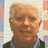Have you created a relationship between your Clients and HerbInventory tables? If not, open the HerbInventory table and select Edit Fields from the tool popup. Then drag the Client reference over from the right column to the center column, as shown at the bottom of the below screenshot. After doing that, open the Clients table and create a button with the following code:

`let v := HerbInventory.'Product Description';`

`alert(v)`

Clicking that button should display the information you requested. However, that might not be necessary because creating the relationship also created a view on the client form of all the Herbs related to that client. See the screenshot below (Clients on top and HerbInventory below):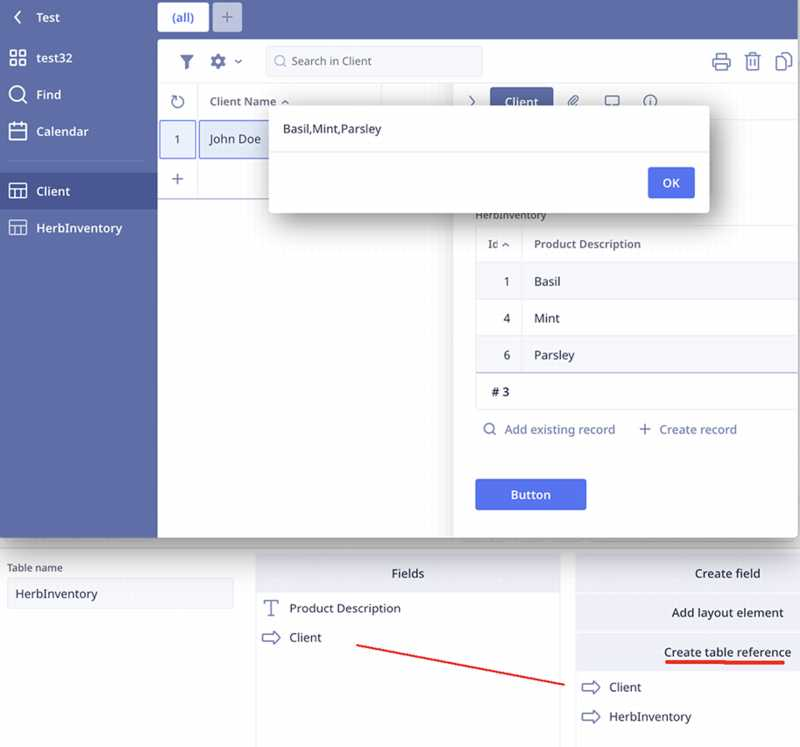Hi Dean,

Thank you for the reply. Yes, currently I have the relationships setup, and everything works. But I still wanted to see if I am able to refer to specific fields in a table using variables, just to have that flexibility available in case I need it later when coding in Ninox. So for example if I had 10 fields named Herb01 all the way to Herb10, I could refer to all 10 using a for loop instead of having to type HerbalInventory.Herb01, HerbalInventory.Herb02 etc. Just wondering if such function exists in the ninox language?

Thanks,

Warren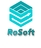No, that is not (yet) possible. Table and field names cannot be addressed via variables.
Steven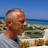You can use an Array. Just read the current record into an array. Then you can access a single field in the record using one variable (via index).Any example code, Kim Schacht?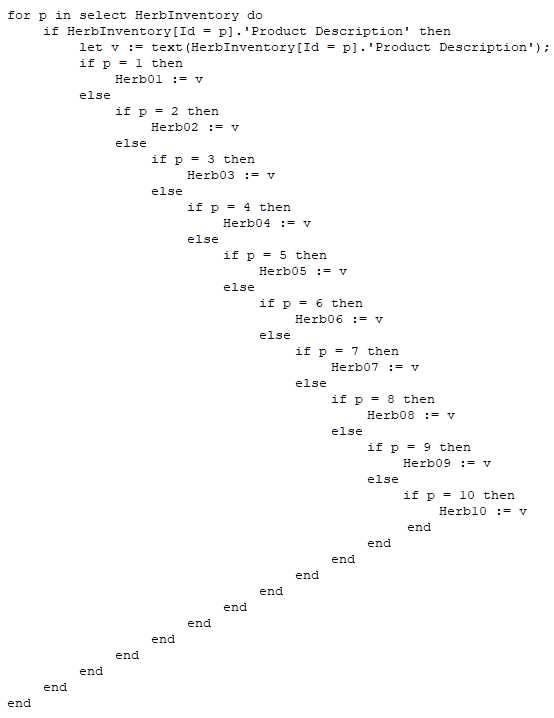Please disregard the above code (I wish there was an undo). Instead, try this:

`let t := HerbInventory.'Product Description';`

`let c := count(t);`
`for p in range(0, c + 11) do`
`  let v := item(t, p - 1);`
`  if p = 1 then`
`    Herb01 := v`
`  else`
`    if p = 2 then`
`      Herb02 := v`
`    else`
`      if p = 3 then`
`        Herb03 := v`
`      else`
`        if p = 4 then`
`          Herb04 := v`
`        else`
`          if p = 5 then`
`            Herb05 := v`
`          else`
`            if p = 6 then`
`              Herb06 := v`
`            else`
`              if p = 7 then`
`                Herb07 := v`
`              else`
`                if p = 8 then`
`                  Herb08 := v`
`                else`
`                  if p = 9 then`
`                    Herb09 := v`
`                  else`
`                    if p = 10 then `
`                      Herb10 := v `
`                    end`
`                  end`
`                end`
`              end`
`            end`
`          end`
`        end`
`      end`
`    end`
`  end`
`end`Line 3 above has a typo. It should be:

`for p in range(0, c + 1) do`